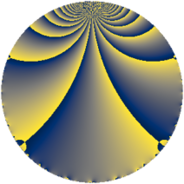# Properties

 Label 70.2.gLevel $70$ Weight $2$ Character orbit 70.g Rep. character $\chi_{70}(13,\cdot)$ Character field $\Q(\zeta_{4})$ Dimension $8$ Newform subspaces $1$ Sturm bound $24$ Trace bound $0$

# Related objects

## Defining parameters

 Level: $$N$$ $$=$$ $$70 = 2 \cdot 5 \cdot 7$$ Weight: $$k$$ $$=$$ $$2$$ Character orbit: $$[\chi]$$ $$=$$ 70.g (of order $$4$$ and degree $$2$$) Character conductor: $$\operatorname{cond}(\chi)$$ $$=$$ $$35$$ Character field: $$\Q(i)$$ Newform subspaces: $$1$$ Sturm bound: $$24$$ Trace bound: $$0$$

## Dimensions

The following table gives the dimensions of various subspaces of $$M_{2}(70, [\chi])$$.

Total New Old
Modular forms 32 8 24
Cusp forms 16 8 8
Eisenstein series 16 0 16

## Trace form

 $$8q - 8q^{7} + O(q^{10})$$ $$8q - 8q^{7} - 16q^{15} - 8q^{16} + 8q^{18} + 16q^{21} - 16q^{22} - 8q^{23} + 8q^{28} + 16q^{30} - 8q^{35} + 8q^{36} + 32q^{37} + 32q^{43} - 16q^{46} + 32q^{50} - 32q^{51} - 32q^{53} - 8q^{56} - 8q^{57} - 16q^{58} - 8q^{60} + 8q^{65} + 16q^{67} - 24q^{70} - 16q^{71} + 8q^{72} - 16q^{77} - 40q^{78} + 24q^{81} - 48q^{85} + 16q^{88} + 16q^{91} - 8q^{92} - 16q^{93} + 64q^{95} + 32q^{98} + O(q^{100})$$

## Decomposition of $$S_{2}^{\mathrm{new}}(70, [\chi])$$ into newform subspaces

Label Dim. $$A$$ Field CM Traces $q$-expansion
$$a_2$$ $$a_3$$ $$a_5$$ $$a_7$$
70.2.g.a $$8$$ $$0.559$$ $$\Q(\zeta_{16})$$ None $$0$$ $$0$$ $$0$$ $$-8$$ $$q-\zeta_{16}^{6}q^{2}+(\zeta_{16}-\zeta_{16}^{3})q^{3}-\zeta_{16}^{4}q^{4}+\cdots$$

## Decomposition of $$S_{2}^{\mathrm{old}}(70, [\chi])$$ into lower level spaces

$$S_{2}^{\mathrm{old}}(70, [\chi]) \cong$$ $$S_{2}^{\mathrm{new}}(35, [\chi])$$$$^{\oplus 2}$$